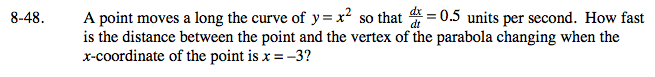### Home > CALC > Chapter 8 > Lesson 8.1.4 > Problem8-48

8-48.Careful! Notice that the derivative is given as change in x with respect to t, not change in y with respect to x.
What was shown was a part of an implicit differentiation problem.

This is a related rates problem.
The question is asking about the rate that the secant line between the moving point and the vertex is
changing at the moment when that moving point is at (-3, 9).

Start by finding the equation of a secant line that connects an arbitrary point (x, x²) with the origin.
You could use the Pythagorean Theorem--note, you do not have to solve for d.

Implicitly differentiate that equation with respect to time.

Also, evaluate the change d with respect to t when x = −3 and the change in x with respect to t equals 0.5.
You will find the rate that the distance will change when the moving point reaches (−3, 9).